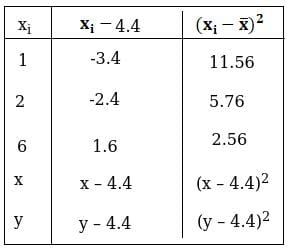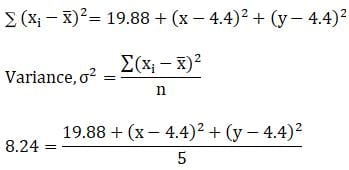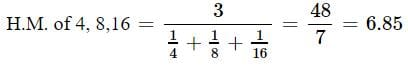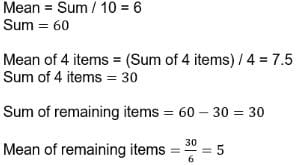# Test: Statistics- 1

## 25 Questions MCQ Test Mathematics For JEE | Test: Statistics- 1

Description
Attempt Test: Statistics- 1 | 25 questions in 25 minutes | Mock test for JEE preparation | Free important questions MCQ to study Mathematics For JEE for JEE Exam | Download free PDF with solutions
QUESTION: 1

### A batsman scores runs in 10 innings 38,70,48,34,42,55,63,46,54 and 44, then the mean deviation is

Solution:

Arranging the given data in ascending order,
we have 34, 38, 42, 44, 46, 48, 54, 55, 63, 70
Here, Median M = (46+48)/2
=47
(∵ n = 10, median is the mean of 5th and 6th items)
∴ Mean deviation = ∑|xi−M|/n
=∑|xi−47|/10
= (13+9+5+3+1+1+7+8+16+23)/10
=8.6

QUESTION: 2

### The S.D. of the observations 22,26,28,20,24,30 is

Solution:

μ = (22 + 26 + 28 + 20 + 24 + 30)/6
= 150/6
= 25
x(i) = (xi - μ)2
x(22) = (22-25)2 = 9
x(26) = (26-25)2 = 1
x(28) = (28-25)2 = 9
x(20) = (20-25)2 = 25
x(24) = (24-25)2 = 1
x(30) = (30-25)2 = 25

(xi - μ)2  = 70
Standard deviation : [(xi - μ)2]/N
= (70/6)½
= 3.42

QUESTION: 3

### If the angle between two lines of regression is 900, then it represents

Solution:
QUESTION: 4

The two lines of regression are 2x - 7y + 6 = 0 and 7x – 2y +1 = 0. What is correlation coefficient between x and y ?

Solution:

ρ = (b(xy) * b(yx))
But sign of ρρ is same as sign of b(xy), b(yx)
Therefore, ρ = 2/7

QUESTION: 5

The two lines of regression are x + 4y = 3 and 3x +y = 15. value of x for y = 3 is

Solution:

Given lines are x+4y=3   (i)
& 3x+y=15        (ii)
We first check which of the line (i) & (ii) is line of regression y
on x and x on y. Let line x+4y=3 be the line of regression x on y, then
other be the line of regression y on x.
∴ From (i) i.e. line of regression x on y we have x=−4y+3
∴ b(xy)=regression coefficient x on y=−4 and 3x+y=15
∴ y=−3x+15
∴ b(yx)=regression coefficient y on x=-3
Now count r2=b(yx)b(xy)=(−4)(−3)=12
⇒ ∣r∣=2(3)1/2
​which is not possible as 0≤r2≤1. So our assumption is wrong
and line x+4y=3 is line of regression y on x & 3x+y=15 is line of
regression x on y.
∴ 3x+y=15
⇒ x=(15−y)/3
⇒ x=(15−3)/3   (By putting y=3)
​= 4

QUESTION: 6

The mean of 5 observations is 4.4 and their variance is 8.24. If three observations are 1,2 and 6, the other two observations are

Solution:

Let the other nos. be a and b
then (x+y+1+2+6)/5 = 4.4
x + y = 13 ---------------------(1)
Variance = 8.2441.2 = 19.88 + (x2 + 19.36 – 8.8x) + (y2 + 19.36 – 8.8y)
21.32 = x2 + y2 + 38.72 – 8.8(x + y)
x2 + y2 + 38.72 – 8.8(13) – 21.32 = 0
(using equation (1))
x2 + y2 – 97 = 0 …(2)
Squaring equation (1) both the sides,
we get (x + y)2 = (13)^2
x2 + y2 + 2xy = 169
97 + 2xy = 169
(using equation (2))
xy = 36 or x = 36/y (1)
⇒ 36/y + y = 13
y2 + 36 = 13y
y2 – 13y + 36 = 0
(y – 4)(y – 9) = 0
Either (y – 4) = 0 or (y – 9) = 0
⇒ y = 4 or y = 9
For y = 4 x = 36/y
= 36/4 = 12
For y = 9
x = 36/9
x = 4
Thus, remaining two observations are 4 and 9.

QUESTION: 7

If x and y are related as y – 4x = 3, then the nature of correlation between x and y is

Solution:

The correlation between x and y is positive correlation because it is a correlation where if one variable increases, the other also increases, and if one variable decreases, the other also decreases.

QUESTION: 8

Coefficient of correlation between the observations (1, 6) , (2 , 5) , (3 , 4) , (4 , 3) , (5 , 2) , (6 , 1) is

Solution:
QUESTION: 9

If the two lines of regression of a bivariate distribution coincide, then the correlation coefficient ρρsatisfies.

Solution:
QUESTION: 10

For a moderately skewed distribution, quartile deviation and the standard deviation are related by

Solution:

The relationship between the mean, quartile and the standard deviation are as follows:
Mean Deviation is the mean of all the absolute deviations of a set of data.
Quartile deviation is the difference between “first and third quartiles” in any distribution.
Standard deviation measures the “dispersion of the data set” that is relative to its mean.
Mean Deviation = 4/5 × Quartile deviation
Standard Deviation = 3/2 × Quartile deviation

QUESTION: 11

Which one of the following average is most affected of extreme observations ?

Solution:
QUESTION: 12

The H.M. of 4,8,16 is

Solution:QUESTION: 13

The Mode of the following items is 0,1,6,7,2,3,7,6,6,2,6,0,5,6,0.

Solution:
QUESTION: 14

The statistical method which helps us to estimate or predict the unknown value of one variable from the known value of the related variable is called

Solution:
QUESTION: 15

The coefficient of correlation r satisfies

Solution:
QUESTION: 16

S.D. of a data is 6. When each observation is increased by 1, then the S.D. of new data is

Solution:
QUESTION: 17

The most stable measure of central tendency is

Solution:
QUESTION: 18

The mean weight of a group of 10 items is 28 and that of another group of n items is 35.The mean of combined group of 10 + n items is found to be 30. The value of n is

Solution:
QUESTION: 19

The measure of variation which is least affected by extreme items is

Solution:
QUESTION: 20

A group of 10 items has mean 6. If the mean of 4 of these items is 7.5, then the mean of the remaining items is

Solution:QUESTION: 21

If the coefficient of variation between x and y is 0.28, covariance between x and y is 7.6, and the variance of x is 9, then the S.D. of the y series is

Solution:

Correlation coefficient = cov (x,y)/ (std deviation (x) ×std deviation (y))
Correlation coefficient  = 0.28
cov (x,y) = 7.6
variance of x is 9.
=> std deviation (x) = √variance  of X = √9 = 3
=>  0.28  = 7.6 / ( 3 * std deviation (y))
=> std deviation (y) = 7.6 / ( 3 * 0.28)
=> std deviation (y) = 9.05
standard deviation of Y series = 9.05

QUESTION: 22

The Q.D. of the daily wages (in Rs) of 7 persons given below: 12,7,15,10,17,19,25 is

Solution:
QUESTION: 23

Which of the following is not a measure of central tendency :

Solution:
QUESTION: 24

If the mean of numbers 27,31,89,107,156 is 82, then the mean of 130,126,68,50,1 is :

Solution:
QUESTION: 25

If in moderately asymmetrical distribution mode and mean of the data are 6 μ and 9 μ respectively, then median is

Solution:

Median = [mode + 2(mean)]/3
= [6μ+2(9μ)]/3
= 24μ/3
= 8μUse Code STAYHOME200 and get INR 200 additional OFF Use Coupon Code